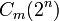# Projective symplectic group

Jump to: navigation, search

## Definition

Let$m$ be a positive integer and$K$ be any field. The projective symplectic group$PSp(2m,k)$ or$PSp_{2m}(K)$ is defined in the following equivalent ways:

• It is the quotient of the symplectic group$Sp(2m,K)$ by the scalar matrices in the group.
• It is the inner automorphism group of the symplectic group$Sp(2m,K)$, i.e., the quotient of that group by its center.

For a prime power$q$, we denote by$PSp(2m,q)$ the group$PSp(2m,\mathbb{F}_q)$ where$\mathbb{F}_q$ is the (unique up to isomorphism) field of size$q$.

### Chevalley notation

The projective symplectic group$PSp(2m,K)$ is the Chevalley group of type C, denoted$C_m(K)$. Note that the degree parameter when describing it as a Chevalley group is half the size of the matrices.

For a prime power$q$, we denote by$C_m(q)$ the group$C_m(\mathbb{F}_q)$ where$\mathbb{F}_q$ is the (unique up to isomorphism) field of size$q$.

## Facts

### Collisions with other Chevalley groups

•$C_1(K)$ is isomorphic to$B_1(K)$ as well as to$A_1(K)$, which is the projective special linear group of degree two$PSL(2,K)$.
•$C_m(2^n)$ is isomorphic to$B_m(2^n)$, where$B_m$ denotes the Chevalley group of type B, and arises as a subgroup of the orthogonal group.

### Simplicity

• Projective symplectic group is simple except the cases$PSp(2,2), PSp(2,3), PSp(4,2)$.